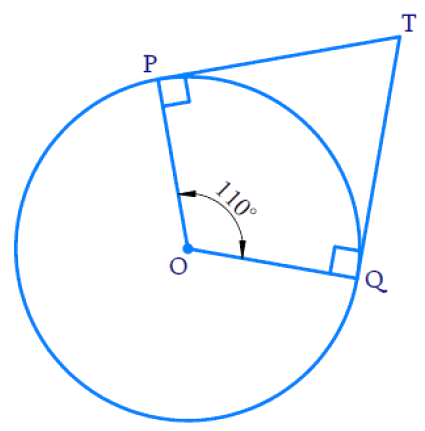# Ex.10.2 Q2 Circles Solution - NCERT Maths Class 10

Go back to  'Ex.10.2'

## Question

In the given figure, if $$TP$$ and $$TQ$$ are the two tangents to a circle with centre $$O$$ so that $$\angle {P O Q} = 110 ^ { \circ }$$, then $$\angle {P T Q}$$ is equal to

(A)$$60^\circ$$

(B)$$70^\circ$$

(C)$$80^\circ$$

(D)$$90^\circ$$Video Solution
Circles
Ex 10.2 | Question 2

## Text Solution

What is Known?

(i) $$TP$$ and $$TQ$$ are tangents to a circle with Centre $$O$$

(ii) $$\angle {P O Q}=110^{\circ}$$

What is Unknown?

$$\angle {PTQ}$$

Reasoning:

• Tangent at any point of a circle is  perpendicular to the radius through the point of contact.
• In the above figure $$OPTQ$$ is a quadrilateral and $$\angle {P}$$ and $$\angle {Q}$$  are $$90^{\circ}$$
• Sum of the angles of a quadrilateral is $$360^{\circ}$$

Steps:

$$\therefore \;$$In $$OPTQ,$$

\begin{align} \left[ \begin{array} & \angle Q+\angle P+ \\ \angle POQ+\angle PTQ \\ \end{array} \right]&\!=\!{{360}^{{}^\circ }} \\ \left[ \begin{array} & {{90}^{{}^\circ }}+{{90}^{{}^\circ }}+ \\ {{110}^{{}^\circ }}+\angle PTQ \\ \end{array} \right]&\!=\!{{360}^{{}^\circ }} \\ {{290}^{{}^\circ }}+\angle PTQ&\!=\!{{360}^{{}^\circ }} \\ \angle PTQ&\!=\!{{360}^{{}^\circ }}-{{290}^{{}^\circ }} \\ \angle PTQ&\!=\!{{70}^{{}^\circ }} \\ \end{align}

Hence the correct Option is B

Learn from the best math teachers and top your exams

• Live one on one classroom and doubt clearing
• Practice worksheets in and after class for conceptual clarity
• Personalized curriculum to keep up with school